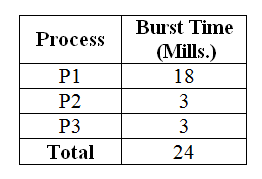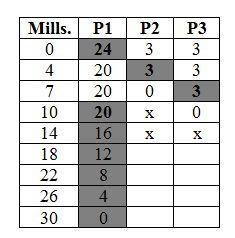• Round – Robin scheduling is one of the oldest, simplest and most widely used algorithm. This algorithm is specially designed for time-sharing system and is similar to FCFS scheduling algorithm. Major difference between FCFS and Round-Robin is that FCFS is non-preemptive while Round-Robin is of preemptive type.
• According to this policy, each process is allocated equal unit of time called a Time Quantum or Time Slice. It is generally from 10-100 millisecond. The ready queue is implemented as a circular queue.
• The CPU scheduler selects process  sequentially from the ready queue and allocate the CPU for 1 time quantum. To implement round-robbing scheduling, the ready queue is implemented as a FIFO queue. New processes are added at the end of the ready queue.
• The CPU scheduler selects the 1st process from the ready queue and sets a timer to interrupt after 1 time quantum. One of the two things will happen

(1) A process may have a CPU burst of less than 1 time quantum.

• In this case the process will release the CPU voluntarily.

(2) If the CPU burst of the currently running process is longer than 1 time quantum, the timer will expire and process will be put at the end of the ready queue for its next turn.

• The CPU scheduler will then select the next process from the ready queue. The avg. waiting time under this policy is often long. In this algorithm no process is allocated the CPU for more than one time quantum. If CPU burst of a process exceeds one time quantum, that process is preempted and is put back in the ready queue.
• Lets try to understand the concept of RR algorithm by the following example.

Consider the following set of process and their burst time. Find out average waiting time and average turnaround time using RR scheduling algorithm.  Time Quantum is of 4 milliseconds. All processes are given in their arrival order.• According to Round-Robin algorithm, processes are given the CPU in sequential manner and each process assign CPU for one time quantum.
• The first process to come is the process P1 so it will get the CPU first for one time quantum and hence waiting time for P1 will be zero. Our time quantum is 4 milliseconds but the burst time of P1 is 18 milliseconds so it can not complete its execution in one time quantum so in first turn it will execute for one time quantum (i.e. 4 milliseconds). As soon as time slice expires, P1 will be put at the end of the ready queue for their next turn. Now in sequential manner, process P2 will get the CPU control after waiting for 4 millisecods. Its burst time is 3 milliseconds which is less than the time quantum so it will execute for 3 milliseconds and after finishing its task it will voluntarily release the CPU. Process P2 will be removed from the memory and next process P3 will get the CPU. Same as P2, process P3 will execute for 3 milliseconds as its burst time is less than the time quantum and eventually will release the CPU control voluntarily.• Since we no more process  are left, the first process P1 will get the CPU in round robin fashion. Recall that it was executed for one time quantum and it has still 14 milliseconds (18-4) of burst time left for execution. As its burst time is still greater than the time quantum, it will execute for one time quantum and since no more process are left, process P1 will continue its turn each time the time slice gets expired.

#### Average Waiting Time

``````Average Waiting Time = Total Waiting Time / No.of Process

Waiting Time = Total Waiting Time - Previous execution

P1 = 10 - 4 = 6
P2 =  3 - 0 = 3
P3 =  3 - 0 = 3
-------------------
Total -->    12 Mills.

A.W.T = 12 / 3

= 4 Mills.

Turnaround Time
P1 = 30
P2 =  7
P3 = 10
----------
Total 47 Mills.

A.T.T = 47/3
=15.66 Mills. ``````